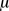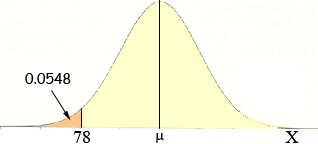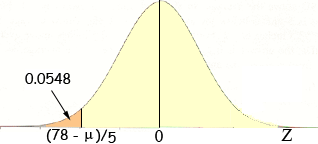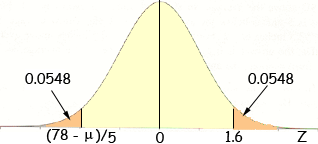In a certain normal distribution, find the mean when the standard deviation is 5 and 5.48% of the area lies to the left of 78. Please help, I am a student and this homework is so confusing. Mary Mary, I think that drawing a diagram helps clarify what is being asked. This is a normal distribution so the curve is "bell-shaped" but you don't know the meanso you can't place the Y-axis. The area under the curve is 1 and the area under the curve to the left of 78 is quite small, 5.48%, that is 0.0548, so 78 must be quite far to the left. I am going to use X for the random variable.To use the area under the curve value, 0.0548, you are going to need to use the standard normal table so you need to convert X to the standard normal variable Z. You know the standard deviation is 5 so the transformation is Z = (X -)/= (X -)/5 and the diagram becomesNow I need to find 0.0548 in the standard normal table. My table reads areas to the right of the value so when I look for 0.0548 I find a Z-value of 1.6Since the normal distribution is symmetric about the mean -1.6 = (78 -)/5 Solve for. Penny